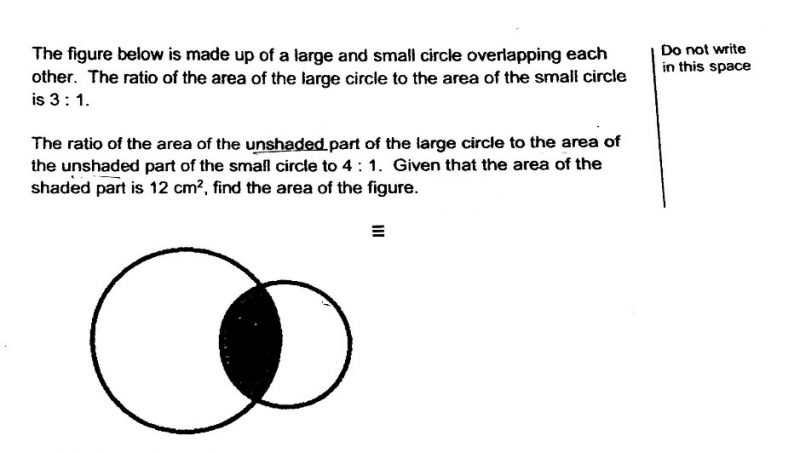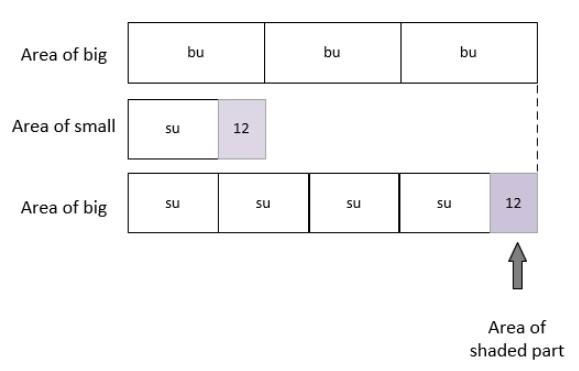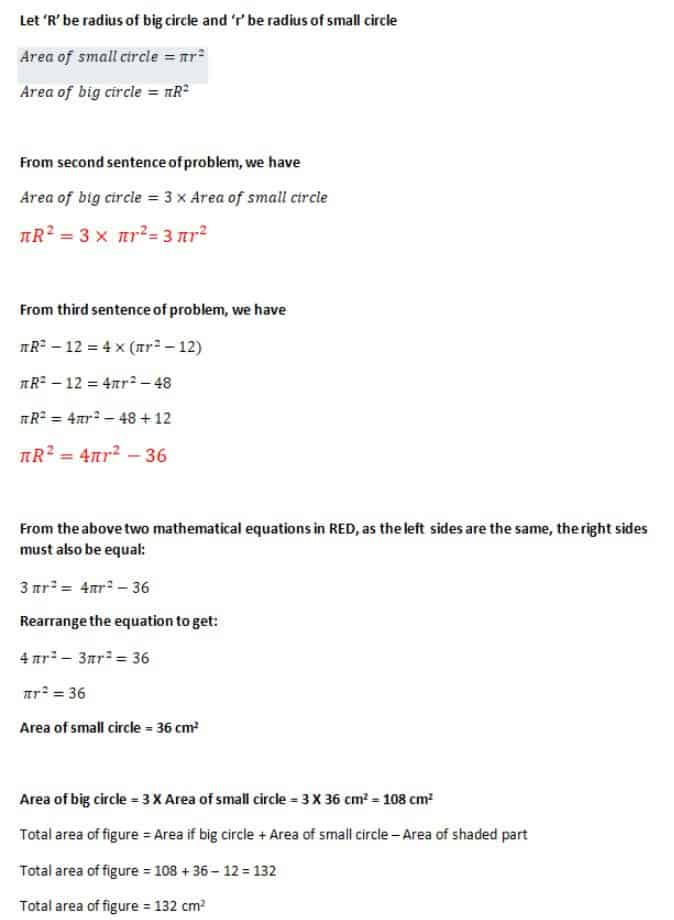QuestionModel diagram Solution:

In the diagram,

‘bu’ stands for big unit

‘su’ stands for small unit

From the model diagram,

Area of big circle ⇒4su+12=3bu

Area of big circle ⇒4su+12=3 x bu

=3 x (su+12)

=3su+36

Therefore we have:

4su+12=3su+36

Rearrange the unknown and known in the above to get:

4su-3su=36-12=24

1su=24

Total area of figure⇒5su+12=5 x 24+12=132

0 Replies 0 Likes0 Replies 0 Likes